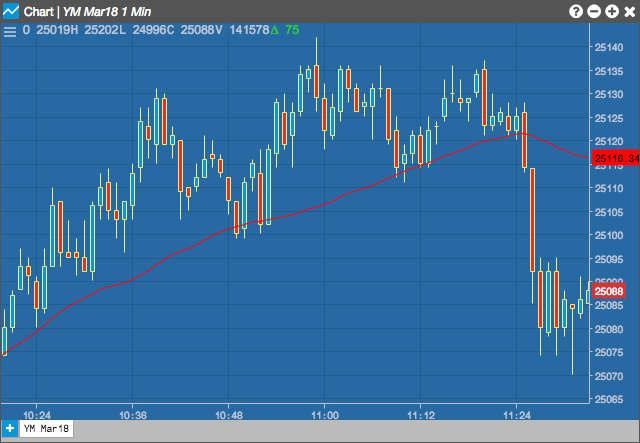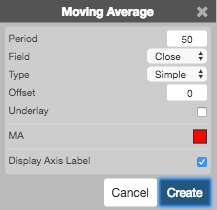Charts

# Moving Average (MA)

A moving average creates a series of averages of different subsets. Each new subset remains a constant length by adding the newest value and removing the oldest. A moving average is defined by the user over n-periods of data. This technical indicator along with several others allow the user to select which type of moving average to use in the calculation. The Formula section shows the formula for each type.

The direction of the moving average (higher, lower, or flat) indicates the trend of the market and its slope indicates the strength of the trend. Longer averages are used to identify longer-term trends while shorter averages are used to identify shorter-term trends## Configuration Options• Period: Number of bars to use in the calculations.
• Field: Price or combination of prices to use as the base for average calculations. Possible values include:
• Open
• High
• Low
• Close
• HL/2 $$\left ( \frac{High + Low}{2} \right )$$
• HLC/3 $$\left ( \frac{High + Low + Close}{3} \right )$$
• HLCC/4 $$\left ( \frac{High + Low + Close + Close}{4} \right )$$
• OHLC/4 $$\left ( \frac{Open + High + Low + Close}{4} \right )$$
• Moving Average Type: Type of moving average to use in the calculations:
• Simple
• Exponential
• Time Series
• Triangular
• Variable
• VIDYA
• Weighted
• Welles Winder
• Hull
• Double Exponential
• Triple Exponential
• Offset:
• Underlay: Whether to display the moving average under the chart.
• Color Selectors: Colors to use for graph elements.
• Display Axis Label: Whether to display the most recent value on the Y axis.

## Formula

$Simple = MA = \frac{\sum_{i=1}^{n} Close_{i}}{n}$

$Exponential = EMA = (Close_{n} - EMA_{t-1}) \times k + EMA_{n-1}$

where k = the smoothing constant, equal to $$\frac{2}{n+1}$$

and n = the number of periods in a simple moving average roughly approximated by the EMA

$Time\;Series = TSMA = \frac{\sum_{i=1}^{n} Close_{i}}{n}$

$Triangular = TMA = \frac{\sum_{i=1}^{n} MA_{i}}{n}$

where $$MA = \frac{\sum_{i=1}^{n} Close_{i}}{n}$$

$Variable = VMA = \frac{P + (a \times b)P1 + {(a \times b)^2}P2 +...+{(a \times b)^{(n-1)}}P(n-1)}{1 + (a \times b) + (a \times b)^2 +...+ (a \times b)^{(n-1)}}$

$Weighted = WMA = \frac{n \times P + {(n-1) \times P1} + {(n-2) \times P2} +...+ P(n-1)}{1 + 2 +3 + ... +n}$

$Welles\;Wilder Smoothing = WWS_{n} = WWS_{n-1} - \left ( \frac{WWS_{n-1}}{n} \right )+(Value_{n})$

where the first calculation of WWS uses a simple moving average $$WWS_{1} = \frac{\sum_{i=1}^{n} Close_{i}}{n}$$